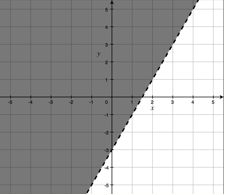## Friday, November 10, 2006

### Next Lesson: Graphing Linear InequalitiesWe are finally moving on from the functions unit into a brief unit on systems of equations and inequalities. Last year, I included linear programming in this unit, because it is a great application not just of systems of inequalities, but being able to think critically about a problem, convert verbal information into a mathematical model, and so forth. Unfortunately, I think this topic will be a casualty of going so deep with functions this year. Every time I don't teach some topic, I feel like I am denying them access to ideas that other students will know. Maybe I can squeeze in a one-lesson overview just so they see the idea.

After the unit test, I gave students a homework to review graphing linear functions (horizontal and vertical, slope-intercept, standard form, finding equations of a line, etc.). I think they were happy to get something that was so "easy" for a change.

In this lesson (I actually taught it yesterday), students first were given a Do Now to explore the idea of shading a solution region. I asked them to graph x - 2y = 8. Then, I gave them a bunch of points and the inequality x - 2y < 8, and asked them to algebraically determine if each point was a solution to the inequality. If it was, they had to plot a Y at the coordinates. If not, they had to plot an N. Seeing all the Ys on one side of the line (and on the line itself) and the Ns on the other helped get across the idea of the solution region. Last year, they were taught to shade the solution region in, but I wanted to make sure that they understood what the shading really means. We talked about how the picture would look different if the inequality symbol flipped, or was exclusively greater or less than.

After we established this concept, I just did some direct instruction and showed them how to graph linear inequalities in various forms: x < c; y < c; |x| < c; |y| < c; y < mx + b; ax + by < c. After each example I did, they did a similar example on their own (switching the type of inequality symbol).

The class ended with some individual/pair practice of graphing. The practice also included determining visually if a point is a solution (given the graph), and then confirming this algebraically.

The homework includes more of the same type of practice. It also has a couple of non-linear inequality challenge problems (like y < (x - 3)^2 + 4) to help them work on their synthesis skills.

I'll post the whole lesson on ILoveMath.org